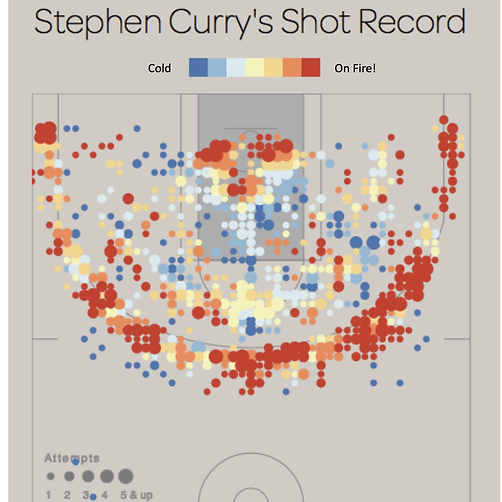top of page## The influence of language on thought. This project involves studying how subtle changes in mathematical language influences students' understanding of fraction multiplication (a well-documented area of difficulty for students). Students (Grades 5-8) will answer fraction multiplication questions that either use the symbol 'x' (e.g., "What's 1/2 x 1/2?") or use the word 'of' (e.g., "What's 1/2 of 1/2?"). We're interested in how this difference might influence one's understanding and visualization of fraction multiplication. This research is part of our lab's larger focus on the role of spatial visualization in mathematical cognition and learning. Note: The image shows data from our pilot study.## What's your relationship with math? This project involves studying elementary school teachers' relationship with mathematics. The goal is to better understand the factors and experiences -- both positive and negative -- that have shaped one's relationship with mathematics over the lifespan. In doing so, we hope to provide new insights into how teachers' mathematical identities are formed and the implications this might have for future generations of students. This project is part of our lab's larger focus on the role of mathematics attitudes (e.g., math anxiety) in mathematics learning and engagement.bottom of page